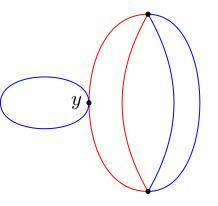# 8.03 Normal covering spaces

Below the video you will find accompanying notes and some pre-class questions.

# Notes

## Example

(0.25) Consider the covering space $$p_n\colon S^1\to S^1$$, $$p_n(z)=z^n$$. What is the deck group of this covering space? Deck transformations are covering isomorphisms $$F\colon(S^1,p_n)\to (S^1,p_n)$$, satisfying $$p_n\circ F=p_n$$. In other words, $(F(z))^n=z^n$ so $$F(z)=\mu z$$ for some $$\mu$$ with $$\mu^n=1$$. The formula $$F(z)=\mu z$$ defines a deck transformation for each $$n$$th root of unity, and any deck transformation must have this form. This tells us that the deck group is $Deck(S^1,p_n)=\mathbf{Z}/n.$

(2.15) If we take $$\pi_1(S^1,1)=\mathbf{Z}$$ and push it forward along $$p_n$$ then we get the subgroup $(p_n)_*\pi_1(S^1,1)=n\mathbf{Z}\subset\pi_1(S^1,1)=\mathbf{Z}.$ Note that, in this example, $Deck(S^1,p_n)\cong\mathbf{Z}/(n\mathbf{Z})=\pi_1(X,x)/p_*\pi_1(Y,y)$ where $$p\colon Y\to X$$ is the covering space.

(3.36) Consider the covering space $$p\colon\mathbf{R}\to S^1$$, $$p(x)=e^{2\pi ix}$$. What is the deck group? It consists of maps $$F\colon\mathbf{R}\to\mathbf{R}$$ such that $$e^{2\pi iF(x)}=e^{2\pi ix}$$, which implies $$F(x)=x+n$$. The deck group is therefore $$Deck(\mathbf{R},p)=\mathbf{Z}$$. The pushforward $$p_*\pi_1(\mathbf{R})$$ is trivial as $$\mathbf{R}$$ is simply-connected, so the quotient $$\pi_1(S^1)/p_*\pi_1(\mathbf{R})$$ is isomorphic to $$\mathbf{Z}$$, which is the deck group.

## Normal subgroups

(5.40) Is this a general phenomenon? No: the subgroup $$p_*\pi_1(Y,y)\subset\pi_1(X,x)$$ might not be a normal subgroup, in which case the quotient $$\pi_1(X,x)/p_*\pi_1(Y,y)$$ doesn't even make sense. However, in the case where $$p_*\pi_1(Y,y)$$ is a normal subgroup of $$\pi_1(X,x)$$, it will turn out to be true that $$Deck(Y,p)=\pi_1(X,x)/p_*\pi_1(Y,y)$$. We single out these covers with a definition:

(6.56) We say that $$p\colon Y\to X$$ is a normal (or regular, or Galois) cover if $$p_*\pi_1(Y,y)$$ is a normal subgroup of $$\pi_1(X,p(y))$$.

Normal covering spaces are maximally symmetric, as we will see from the following lemma.

(7.34) A path-connected covering space $$p\colon Y\to X$$ is normal if and only if $$Deck(Y,p)$$ acts transitively on $$p^{-1}(x)$$ for some $$x\in X$$.

(8.45) Note that $$Deck(Y,p)$$ always acts on $$p^{-1}(x)$$: if $$F\in Deck(Y,p)$$ and $$y\in p^{-1}(x)$$ then $$p(F(y))=p(y)=x$$, so $$F(y)\in p^{-1}(x)$$.

(9.56) Moreover, $$F\in Deck(Y,p)$$ is determined uniquely by its value on a particular point $$y\in p^{-1}(x)$$: indeed, any covering transformation is determined uniquely by its value at a point. Therefore, if the action of $$Deck(Y,p)$$ is transitive on $$p^{-1}(x)$$, then $$Deck(Y,p)$$ is as big as it could possibly be: if it were any bigger, two of its elements would necessarily agree at some point $$y\in p^{-1}(x)$$, and hence would be equal.

(12.29) In our earlier examples, the deck group acts transitively. For $$p_n\colon S^1\to S^1$$, the $$n$$th roots of unity act transitively by rotation on $$p_n^{-1}(1)$$, which it itself the set of $$n$$th roots of unity. For $$p\colon\mathbf{R}\to S^1$$, the group $$\mathbf{Z}$$ acts transitively by translation on $$p^{-1}(1)=\mathbf{Z}$$.

(14.03) Below is a covering space of the figure 8 which is not normal.Let $$x$$ be the cross-point of the figure 8. It has three pre-images, but only one of these (say $$y$$) is the endpoint of a blue loop. Since deck transformations are symmetries of the covering space, they must preserve the unique blue loop, and hence fix this point $$y$$. If a deck transformation fixes a point then it is equal to the identity, by uniqueness. Therefore the deck group in this case is trivial.

## Proof of lemma

(16.57) Assume that $$Deck(Y,p)$$ acts transitively on $$p^{-1}(x)$$. Pick $$\beta\in\pi_1(X,x)$$. We want to show that $\beta p_*\pi_1(Y,y)\beta^{-1}=p_*\pi_1(Y,y).$ We know from an exercise in an earlier class that $\beta p_*\pi_1(Y,y)\beta^{-1}=p_*\pi_1(Y,\sigma_\beta(y)),$ where $$\sigma_\beta\colon p^{-1}(x)\to p^{-1}(x)$$ is the monodromy around $$\beta$$. Therefore it suffices to prove that $$p_*\pi_1(Y,y)=p_*\pi_1(Y,\sigma_\beta(y))$$.

(19.34) By assumption, $$Deck(Y,p)$$ acts transitively, so there exists a deck transformation $$F\colon Y\to Y$$ such that $$F(y)=\sigma_\beta(y)$$ and deck transformations are homeomorphisms, so $(F_*\colon\pi_1(Y,y)\to\pi_1(Y,\sigma_\beta(y))$ is an isomorphism. We also know that $$p\circ F=p$$, so $$p_*\circ F_*=p_*$$. Putting all this together gives: \begin{align*} \beta p_*\pi_1(Y,y)\beta^{-1}&= p_*\pi_1(Y,\sigma_\beta(y))\\ &=p_*(F_*(\pi_1(Y,y)))\\ &=p_*\pi_1(Y,y). \end{align*} Since this holds for all $$\beta\in\pi_1(X,x)$$, this shows that $$p_*\pi_1(Y,y)\subset\pi_1(X,x)$$ is normal.

(21.50) Conversely, suppose that $$p_*\pi_1(Y,y)$$ is a normal subgroup. Let $$y'$$ be another point in $$p^{-1}(x)$$ and let $$\tilde{\beta}$$ be a path in $$Y$$ from $$y$$ to $$y'$$. Then $$y'=\sigma_\beta(y)$$ and $p_*\pi_1(Y,y')=\beta p_*\pi_1(Y,y)\beta^{-1}.$ Since $$p_*\pi_1(Y,y)$$ is normal, we get $$p_*\pi_1(Y,y')=p_*\pi_1(Y,y)$$, so the existence theorem for covering isomorphisms implies that there exists a covering transformation $$F\colon Y\to Y$$ such that $$F(y)=y'$$. Therefore $$Deck(Y,p)$$ acts transitively on $$p^{-1}(x)$$.

# Pre-class questions

1. Consider the non-normal covering space example. What is the subgroup $$p_*\pi_1(Y,y)\subset\pi_1(X,x)$$? Can you see it is not normal without appealing to the lemma we used?